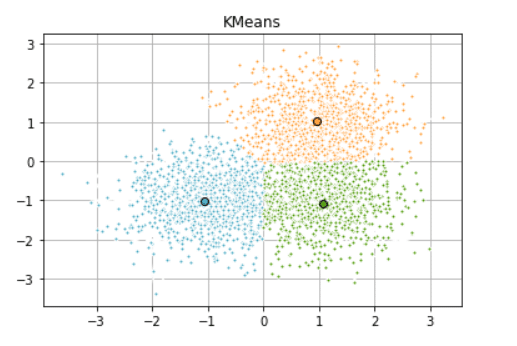# k-means clustering | Python Unsupervised Learning -1

In this series of articles, I will explain the topic of Unsupervised Learning and make examples of it.

Unsupervised learning is a class of machine learning techniques for discovering patterns in data.

For instance, finding the natural “clusters” of customers based on their purchase histories, or searching for patterns and correlations among the purchases and using these patterns to express the data in compressed form.

• Unsupervised learning finds patterns in data
• E.g., clustering customers by their purchases
• Compressing the data using purchases  patterns (dimension reduction)

### Supervised  vs Unsupervised learning

• Supervised learning finds patterns for a prediction task
• E.g., classify tumors as benign or cancerous (labels)
• Unsupervised learning finds patterns in data
• … but without a specific prediction  task in mind

## k-means clustering | Python Unsupervised Learning -1

In this part we’ll cluster some sample data using k-means clustering.

• Finds clusters of samples
• Numbers of clusters must be specified
• Implemented in sklearn (scikit-learn)

Let’s do a simple example, generate sample data and 2D points

We have to import the Iris dataset and other necessary libraries.

```import matplotlib.pyplot as plt
from sklearn import datasets
from sklearn.cluster import KMeans
import pandas as pd
import numpy as np
from sklearn.datasets.samples_generator import make_blobs```

Generate sample data

```np.random.seed(0)

batch_size = 45
centers = [[1, 1], [-1, -1], [1, -1]]
n_clusters = len(centers)
X, labels_true = make_blobs(n_samples=3000, centers=centers, cluster_std=0.7)```

Compute clustering with Means

```k_means = KMeans(n_clusters=3)
k_means.fit(X)
k_means_labels = k_means.labels_
k_means_cluster_centers = k_means.cluster_centers_
k_means_labels_unique = np.unique(k_means_labels)```

Plot result

```colors = ['#4EACC5', '#FF9C34', '#4E9A06']
plt.figure()

for k, col in zip(range(n_clusters), colors):
my_members = k_means_labels == k
cluster_center = k_means_cluster_centers[k]
plt.plot(X[my_members, 0], X[my_members, 1], 'w',
markerfacecolor=col, marker='.')
plt.plot(cluster_center, cluster_center, 'o', markerfacecolor=col,
markeredgecolor='k', markersize=6)
plt.title('KMeans')
plt.grid(True)
plt.show()
```

As a result, you will see a printout like in the picture.Now that we have briefly got to know the k-means algorithm and explained the topic of Unsupervised learning, see you in the next article.

Thank you.

Evaluating a Clustering | Python Unsupervised Learning -2

2,685 views last month,  1 views today